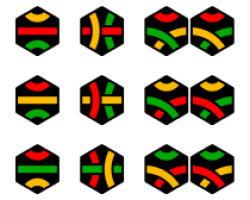# Discrete MathematicsAlgebra includes the study of groups, rings, fields, and vector spaces which are the basic frameworks from which to study many other areas of mathematics and science. Combinatorics is the study of counting the number of possibilities that meet a certain criteria. This subject closely relates to probability.

### Our areas of expertise

Enumerative and algebraic combinatorics, topological combinatorics, Catlan objects, graph theory (extremal graphs), graph colorings, partitions, distributed algorithms, and discrete geometry.